## Logarithms Unmuddled

I often write about topics that I myself have struggled to understand. If I’ve struggled, I assume that many others have struggled as well. Over the years, I’ve found several mathematical concepts confusing, not because I’m mathematically disinclined or disinterested, but because my formal training in mathematics was rather limited and, in some cases, poorly taught. My formal training consisted solely of basic arithmetic in elementary school, basic algebra in middle school, basic geometry in high school, and an introductory statistics course in undergraduate school. When I was in school, I didn’t recognize the value of mathematics—at least not for my life. Later, once I became a data professional, a career that I stumbled into without much planning or preparation, I learned mathematical concepts on my own and on the run whenever the need arose. That wasn’t always easy, and it occasionally led to confusion. Like many mathematical topics, logarithms can be confusing, and they’re rarely explained in clear and accessible terms. How logarithms relate to logarithmic scales and logarithmic growth isn’t at all obvious. In this article, I’ll do my best to cut through the confusion.

Until recently, my understanding (and misunderstanding) of logarithms stemmed from limited encounters with the concept in my work. As a data professional who specialized in data visualization, my knowledge of logarithms consisted primarily of three facts:

1. Along logarithmic scales, each labeled value that typically appears along the scale is a consistent multiple of the previous value (e.g., multiples of 10 resulting in a scale such as 1, 10, 100, 1,000, 10,000, etc.).
2. Logarithmic scales make it easy to compare rates of change in line graphs because equal slopes represent equal rates of change.
3. Logarithmic growth exhibits a pattern that goes up by a constantly decreasing amount.

If you, like me, became involved in data sensemaking (a.k.a., data analysis, business intelligence, analytics, data science, so-called Big Data, etc.) with a meagre foundation in mathematics, your understanding of logarithms might be similar to mine—similarly limited and confused. For example, if you think that the sequence of values 1, 10, 100, 1,000, 10,000, and so on is a sequence of logarithms, you’re mistaken, and should definitely read on.

Before reading on, however, I invite you to take a few minutes to write a definition for each of the following concepts:

• Logarithm
• Logarithmic scale
• Logarithmic growth

In addition to definitions, take some time to describe how these concepts relate to one another. For example, how does a logarithmic scale relate to logarithmic growth? Give it a shot now before reading any further.

Regardless of how much you struggle to define these concepts and their relationships to one another, it’s useful to prime your brain for the topic. Now that you have, let’s dive in.

Logarithms

The logarithm (a.k.a., log) of a number is the power that the log’s base must be raised to equal that number. I realize this definition might not seem clear but hang in here with me. I promise that greater clarity will emerge. Logarithms always have a base (i.e., a number on which it is based). The most common base is 10, expressed as log10, but any number may serve as the base. To determine the log10 value of the number 100, we must determine the power of 10 that equals 100. What this means will become clear in a moment through an example, but before getting to that, let’s review what raising the power of a number means in mathematics.

Raising a number to a power involves multiplying the number by itself a specific number of times. The power indicates how many instances of the number are multiplied. For example, 10 to the power of 3, written as 103 (the 3 in this case is called the exponent), involves multiplying 10 * 10 * 10, which equals 1,000. Raising a number to the power of 1 involves only one instance of that number—there is nothing to multiply—so the number remains unchanged. For example, 101 remains 10. Raising a number to the power of 2 involves multiplying two instances of that number, so 102 is 10 * 10, which equals 100. In these examples so far, the only time multiplication wasn’t involved was with the power of 1. Multiplication is also not involved whenever the exponent is zero or negative. In those cases, raising the power of a number involves division. For example, with the power of 0, rather than multiplying instances of the number by itself, we divide the number by itself, so 100 is equal to 1, for 10 / 10 = 1. Here’s a list of values that result from raising the number 10 to the powers of 0 through 6: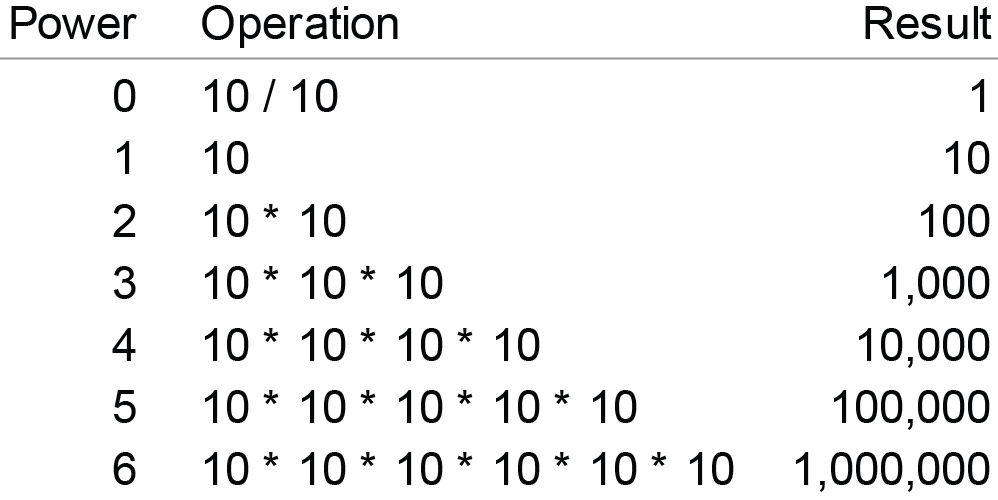Now that we’ve reviewed what it means to raise a number to a particular power, we can get back to logarithms. Remember that the log of a number is the power that the log’s base must be raised to equal that number. So, to find the log2 value of the number 8, we must determine the power of 2 (the log’s base) that is equal to 8. In other words, we must determine how many times 2 must be multiplied by itself to equal 8. Since 21 = 2 and 22 = 4 (i.e., 2 * 2 = 4) and 23 = 8 (i.e., 2 * 2 * 2 = 8), we know that log2 of 8 is 3. Given this procedure, what is the log10 value of 100? It is 2, for 10 must be raised to the power of 2 (i.e., 10 * 10) to equal 100. What’s the log2 of 64? It is 6, for 2 must be raised to the power of 6 (i.e., 2 * 2 * 2 * 2 * 2 * 2) to equal 64.

So far, we’ve only dealt with logs that result in nice, round numbers, but that isn’t always the case. For example, what is the log2 of 100? The log2 of 64 is 6 and the log2 of 128 is 7, so the log2 of 100 is somewhere between 6 and 7. When expressed to eight decimal places, the log2 of 100 is precisely 6.64385619. What is the log10 of 5? It must be less than 1, because 5 is less than 10. The precise answer is 0.698970004.

Have you ever examined a list of the logarithms associated with an incremental sequence of numbers? Doing this is revealing. Here’s a list of the log2 values for the numbers 1 through 32, with an additional column that shows the proportional relationship between log2 values and the numbers on which they’re based: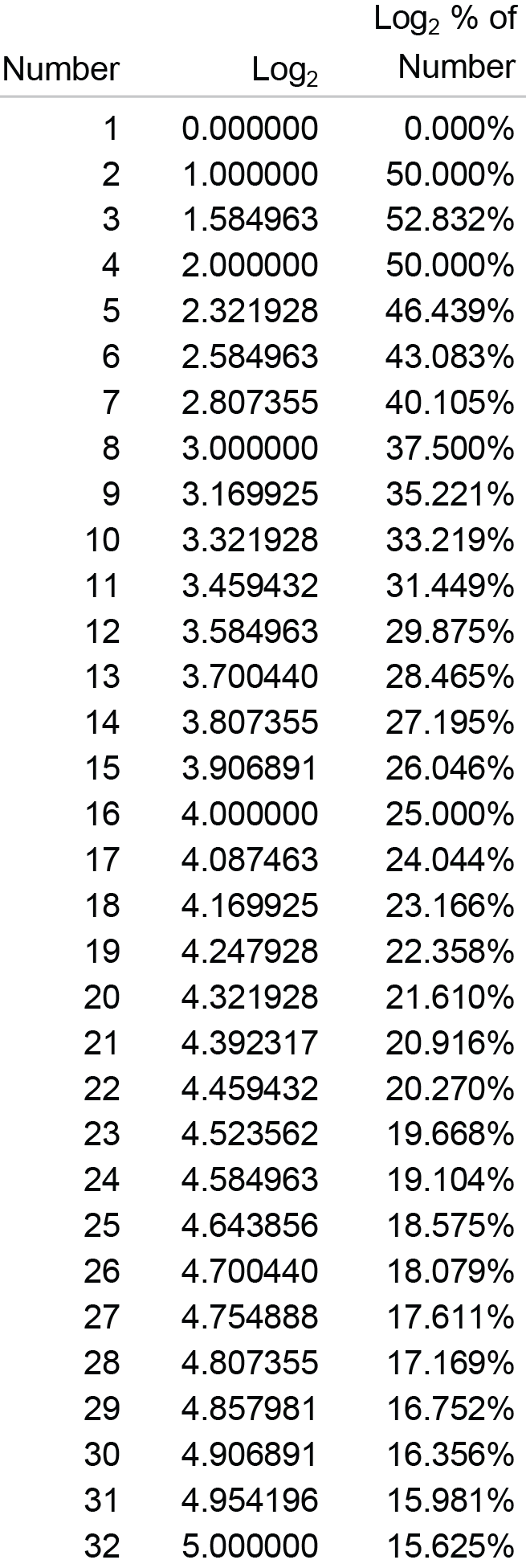Notice that, other than the log2 value of the number 3 (i.e., 1.584963, which is 52.832% of 3), as we read down the list, each log is a decreasing percentage of the number on which it is based. Keep this fact in mind. It will come in handy as we examine logarithmic scales and logarithmic growth.

Logarithmic Scales

A logarithmic scale (a.k.a., log scale) is one in which equal distances along the scale correspond to equal logarithmic distances. Because of the nature of logarithms, each number that typically appears along the scale is a consistent multiple of the previous number. The example below includes a log10 scale along the Y axis.Along a log10 scale, because the base is 10, each number is 10 times the previous number. The example above begins at 1, but it could begin at any number. For example, a log10 scale could begin at 40 and continue with 400, 4,000, 40,000, and so on, each ten times the previous. A log2 scale that begins with 1 would continue with 2, 4, 8, 16, and so on, each two times the previous. Unlike a linear scale in which the intervals from one number to the next are always equal in value, such as 0, 10, 20, 30, 40, etc., along a log scale the intervals (i.e., the quantitative distances between the labeled values) consistently increase in value, each time multiplied by the base.

The numbers 1, 10, 100, 1,000, 10,000, 100,000, and 1,000,000 in the graph above correspond to logarithms with a base of 10, but those numbers are not themselves logarithms. Instead, they are the numbers from which the logarithms were derived. Here’s the scale that appears along the Y axis of the graph above, this time with the actual log10 values 0 through 6 labeled in addition to the numbers 1 through 1,000,000 from which those logarithms were derived.We usually label the log scales with the numbers from which the logarithms were derived rather than the logarithms themselves because the former are typically more familiar and useful.

Another characteristic of a log scale that reinforces its nature bears mentioning, which I’ll illustrate below by featuring a single interval only along the Y axis of the graph shown previously.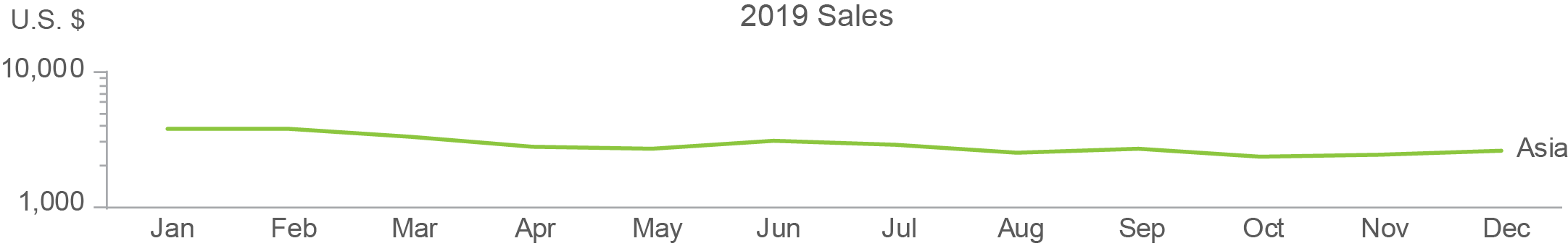Notice that the minor tick marks between 1,000 and 10,000 get closer and closer together from bottom to top. This is easier to see if the scale is enlarged and the minor tick marks are labeled, as I’ve done below.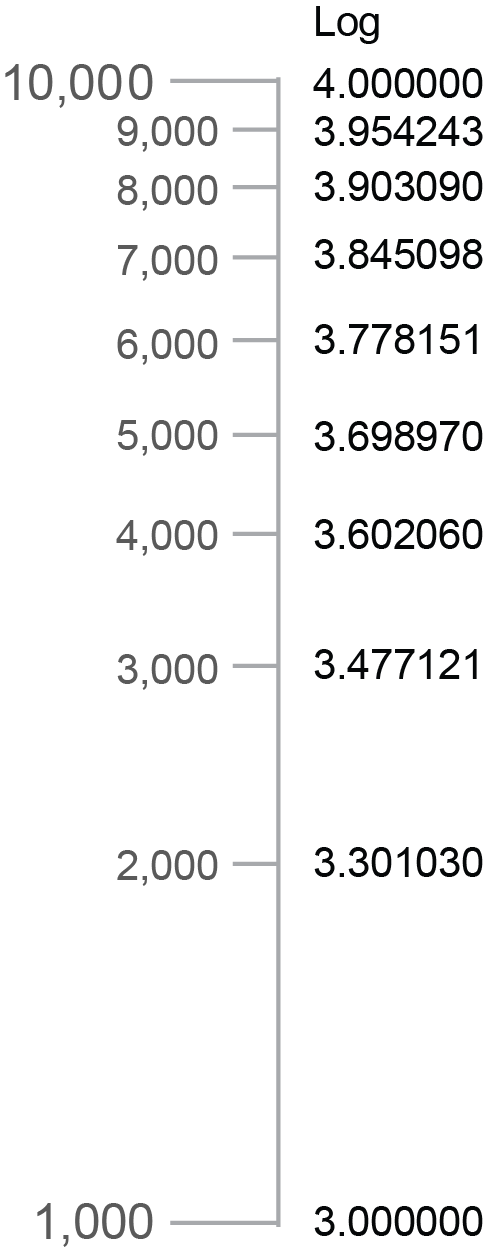Each interval from one tick mark to the next (1,000 to 2,000, 2,000 to 3,000, etc.) consistently covers a numeric range of 1,000, but the spaces between the marks get smaller and smaller because the differences in the logarithms corresponding to those numbers get smaller and smaller. To illustrate this, I included a column of the log10 values that correspond to each tick mark in the example above. The decreasing distances between the tick marks correspond precisely to the decreasing differences between the log values.

Logarithmic Growth

Because the numbers that typically appear as labels on a log scale are each a consistent multiple of the previous number, if you didn’t already understand logarithms, you might assume that logarithmic growth involves a series of values that are each a consistent multiple of the previous value. Here’s an example of how that might look as a series of values: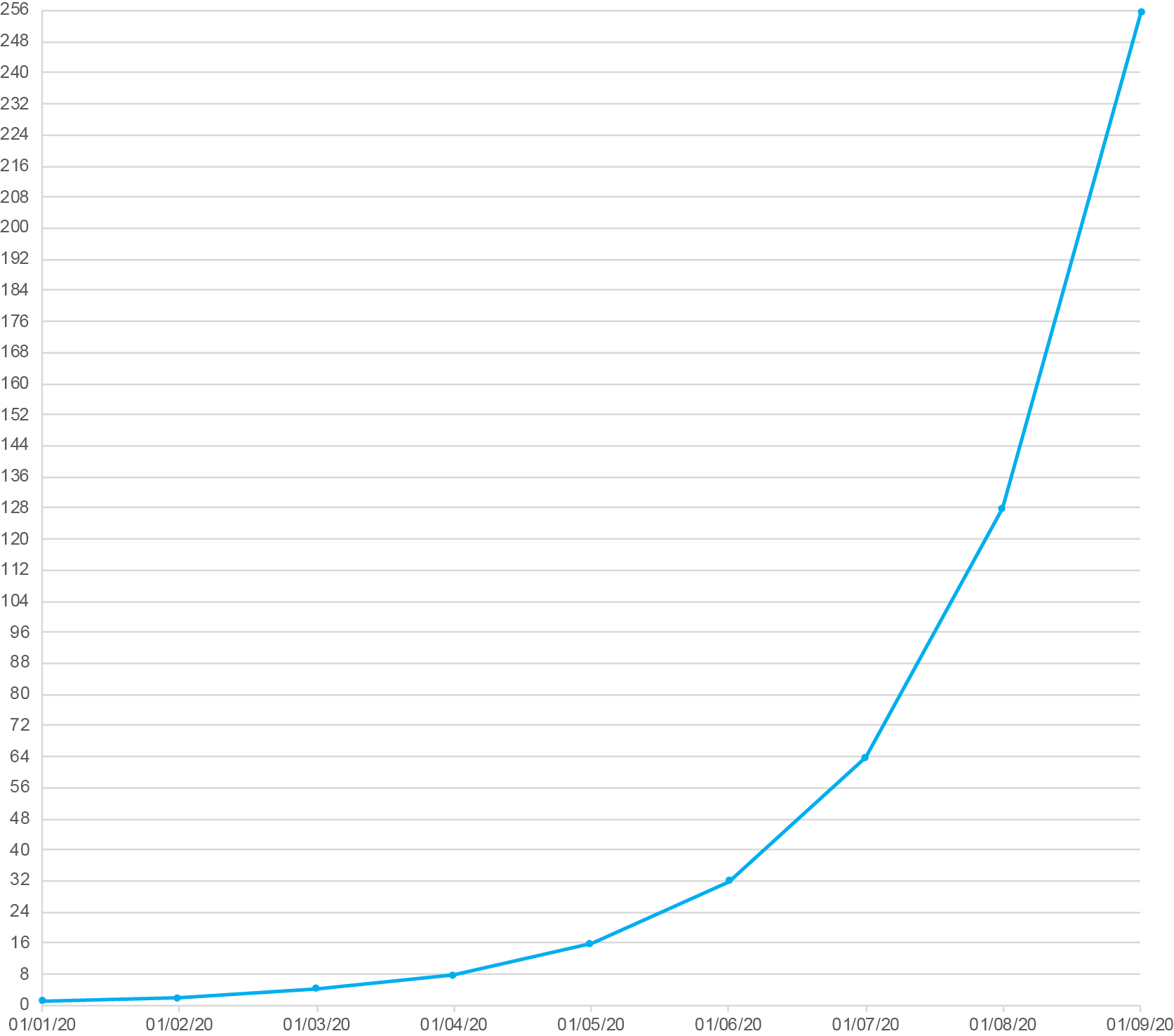In this example, each daily value doubles the previous value. This, however, is not an example of logarithmic growth. It is instead an example of exponential growth (a.k.a., compound growth). With exponential growth, the amount of increase from one value to the next is always greater. Compound interest earned on money in a savings account is an example of exponential growth. As the balance grows, even though the rate of interest remains constant, the amount of growth in dollars consistently increases because of the growing balance. For example, 10% interest on \$100 (i.e., \$10) would increase the balance to \$110 during the first period, and then during the next period, it would be based on \$110 resulting in \$11 of interest, a dollar more. Even though the interest rate remains constant, because the balance grows from one period to the next, the amount of increase grows as well.

Contrary to exponential growth, logarithmic growth (a.k.a., log growth) exhibits a constant decrease in the amount of growth from one value to the next. In other words, it always grows but it does so to a decreasing degree over time. A simple example is the distance that a bullet travels when you shoot it straight up into the air from the moment it leaves the gun until it reaches its apex, before beginning its descent. The height of the bullet starts off by increasing quickly but those increases constantly decrease in amount from one interval of time to the next due to the pull of gravity.

So, how does log growth relate to log scales? It’s not at all obvious, is it? Good luck finding an explanation on the web that’s understandable if you’re not fluent in mathematics. Here’s a graphical example of log growth, based on the log2 values for the numbers 1 through 64: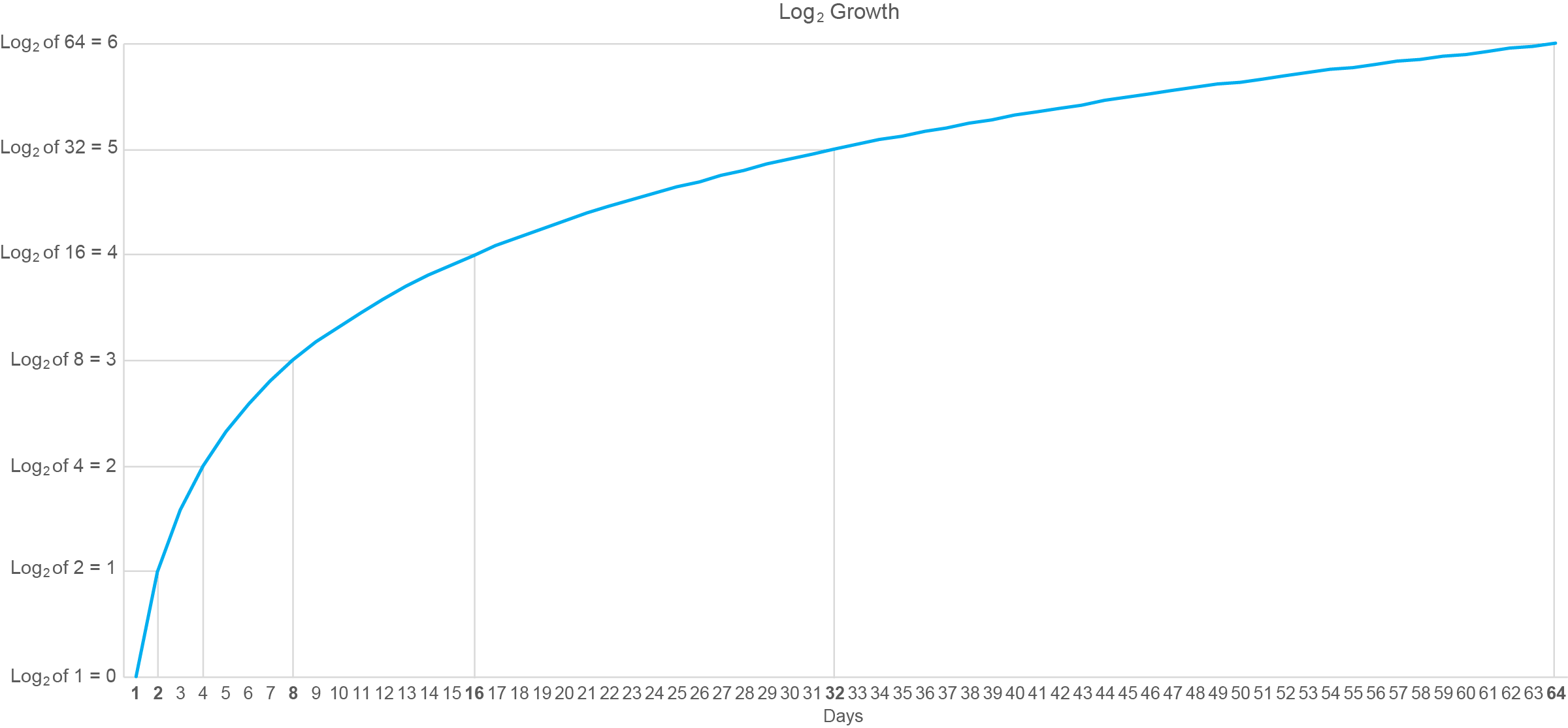I’ve annotated this graph with lines connected to points in time when the logarithm has increased by a whole unit (i.e., from 0 to 1, 1 to 2, etc.). Starting on day 1 the log value is zero and whole-unit increases are subsequently reached on days 2, 4, 8, 16, 32, and 64. Do you recognize this pattern of days along the X axis? It matches the numbers that would appear along a log2 scale that begins with 1. In other words, the intervals between the days on which the logarithms increased by a whole unit consistently grew by a multiple of 2.

Have you noticed that the pattern formed by log growth is the inverse (i.e., flipped top to bottom and left to right version) of the pattern formed by exponential growth? To illustrate this, the graph below displays three different patterns of growth: logarithmic, linear, and exponential.This inverted relationship between patterns of logarithmic and exponential growth visually confirms the inverted relationship that exists between logarithms and the exponential powers that are used to produce them.

Given the nature of logarithms, what do you think would happen to the shape of the blue exponential line above if I changed the scale along the Y axis from linear to logarithmic? If your answer is that the blue line would now take on the shape of logarithmic growth similar to the orange line above, you’re thinking in the right direction, but you went too far. The nature of logarithms to progressively decrease in the amount that they grow from one to the next would cancel out the nature of exponents to progressively increase in amount that they grow from one to the next, resulting in a linear pattern similar to the gray line in the graph above.

I hope you agree that these concepts actually make sense when they’re explained with clear words and examples. You still might not have much use for logarithms unless your work involves advanced mathematics, but now you’re less likely to embarrass yourself by saying something dumb about them, as I’ve done on occasion.

### One Comment on “Logarithms Unmuddled”

By Lorenzo. April 3rd, 2020 at 8:34 am

As it happens, I was just trying to unmuddle this myself! Thank you, Stephen, for all you do with visual communication! Working in business processes, I see visual challenges all the time. Your work and site and workshops (former attendee) are invaluable.